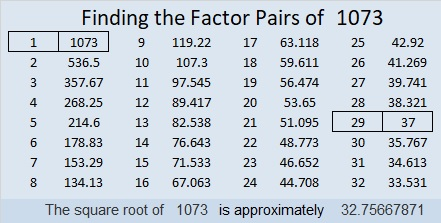# 1073 and Level 1

You might not recognize it, but this puzzle is just a multiplication table. The factors in the table are all missing, and they aren’t in their usual places, but you have everything you need here to find the factors from 1 to 10 and then complete the entire multiplication table. It’s a level 1 puzzle so I am absolutely sure you can solve it!Print the puzzles or type the solution in this excel file: 10-factors-1073-1079

Here is a little information about the number 1073:

• 1073 is a composite number.
• Prime factorization: 1073 = 29 × 37
• The exponents in the prime factorization are 1 and 1. Adding one to each and multiplying we get (1 + 1)(1 + 1) = 2 × 2 = 4. Therefore 1073 has exactly 4 factors.
• Factors of 1073: 1, 29, 37, 1073
• Factor pairs: 1073 = 1 × 1073 or 29 × 37
• 1073 has no square factors that allow its square root to be simplified. √1073 ≈ 32.7566832² + 7² = 1073
28² + 17² = 1073

Because 29 × 37 = 1073, it is the hypotenuse of FOUR Pythagorean triples, two of which are primitives:
348-1015-1073 which is 29 times (12-35-37)
740-777-1073 which is (20-21-29) times 37
448-975-1073 calculated from 2(32)(7), 32² – 7², 32² + 7²
495-952-1073 calculated from 28² – 17², 2(28)(17), 28² + 17²

1073 looks interesting when it is written in some other bases:
It’s 4545 in BASE 6 because 4(6³) + 5(6²) + 4(6) + 5(1) = 1073,
292 in BASE 21 because 2(21²) + 9(21) + 2(1) = 1073, and
TT in BASE 36 (T is 29 base 10) because 29(36) + 29(1) = 29(37) = 1073